Practice Test: Civil Engineering (CE)- 1

# Practice Test: Civil Engineering (CE)- 1

Test Description

## 65 Questions MCQ Test GATE Civil Engineering (CE) 2023 Mock Test Series | Practice Test: Civil Engineering (CE)- 1

Practice Test: Civil Engineering (CE)- 1 for Civil Engineering (CE) 2023 is part of GATE Civil Engineering (CE) 2023 Mock Test Series preparation. The Practice Test: Civil Engineering (CE)- 1 questions and answers have been prepared according to the Civil Engineering (CE) exam syllabus.The Practice Test: Civil Engineering (CE)- 1 MCQs are made for Civil Engineering (CE) 2023 Exam. Find important definitions, questions, notes, meanings, examples, exercises, MCQs and online tests for Practice Test: Civil Engineering (CE)- 1 below.
Solutions of Practice Test: Civil Engineering (CE)- 1 questions in English are available as part of our GATE Civil Engineering (CE) 2023 Mock Test Series for Civil Engineering (CE) & Practice Test: Civil Engineering (CE)- 1 solutions in Hindi for GATE Civil Engineering (CE) 2023 Mock Test Series course. Download more important topics, notes, lectures and mock test series for Civil Engineering (CE) Exam by signing up for free. Attempt Practice Test: Civil Engineering (CE)- 1 | 65 questions in 180 minutes | Mock test for Civil Engineering (CE) preparation | Free important questions MCQ to study GATE Civil Engineering (CE) 2023 Mock Test Series for Civil Engineering (CE) Exam | Download free PDF with solutions
 1 Crore+ students have signed up on EduRev. Have you?
Practice Test: Civil Engineering (CE)- 1 - Question 1

### In each question below are given two statements followed by two conclusions numbered I and II. You have to take the given two statements to be true even if they seem to be at variance from commonly known facts. Read the conclusion and then decide which of the given conclusions logically follows from the two given statements, disregarding commonly known facts. Give answer: (A) If only conclusion I follows (B) If only conclusion II follows (C) If either I or II follows (D) If neither I nor II follows and (E) If both I and II follow. Statements: All bags are cakes. All lamps are cakes. Conclusions: Some lamps are bags. No lamp is bag.

Detailed Solution for Practice Test: Civil Engineering (CE)- 1 - Question 1

Since the middle term 'cakes' is not distributed even once in the premises, no definite conclusion follows. However, I and II involve only the extreme terms and form a complementary pair. So, either I or II follows.

Practice Test: Civil Engineering (CE)- 1 - Question 2

### Man does not live by __________ alone.

Detailed Solution for Practice Test: Civil Engineering (CE)- 1 - Question 2

Man does not live by bread alone.

Practice Test: Civil Engineering (CE)- 1 - Question 3

### Extreme focus on syllabus and studying for tests has become such a dominant concern of Indian students that they close their minds to anything ___________ to the requirements of the exam.

Detailed Solution for Practice Test: Civil Engineering (CE)- 1 - Question 3

Extraneous – irrelevant or unrelated to the subject being dealt with

Practice Test: Civil Engineering (CE)- 1 - Question 4

Select the pair that best expresses a relationship similar to that expressed in the pair:
LIGHT : BLIND

Detailed Solution for Practice Test: Civil Engineering (CE)- 1 - Question 4

Light cannot be seen by blind.
On the similar logic, Speech cannot be spoken by dumb. Sound cannot be heard by deaf.

Practice Test: Civil Engineering (CE)- 1 - Question 5

If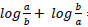= log(a+b), then:

Detailed Solution for Practice Test: Civil Engineering (CE)- 1 - Question 5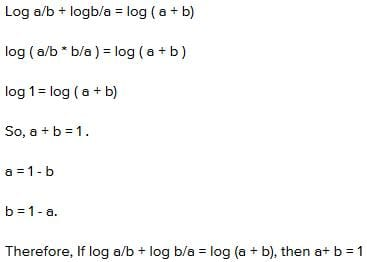Practice Test: Civil Engineering (CE)- 1 - Question 6

In an exam, the average was found to be 50 marks. After deducting computational errors the marks of the 100 candidates had to be changed from 90 to 60 each and average came down  to 45 marks. Total No of candidates who took the exam were.

Detailed Solution for Practice Test: Civil Engineering (CE)- 1 - Question 6

Let number of candidates be n
50 × n – 100 × 30 = 45 × n
n = 600

Practice Test: Civil Engineering (CE)- 1 - Question 7

A solid 4cm cube of wood is coated with red paint on all the six sides. Then the cube is cut into smaller 1cm cubes. How many of these 1cm cubes have no colour on any side?

Detailed Solution for Practice Test: Civil Engineering (CE)- 1 - Question 7

Number of cubes with 3 faces red = 8
Number of cubes with 2 faces red = 24
Number of cubes with 1 face red = 24
Number of cubes with no face red = 64-(24+24+8) = 8

Practice Test: Civil Engineering (CE)- 1 - Question 8

In deriving the equation for the hydraulic jump in a rectangular channel in terms of the conjugate depths and the initial Froude number.

Detailed Solution for Practice Test: Civil Engineering (CE)- 1 - Question 8

Continuity equation and momentum equation are used for the hydraulic jump in terms of the conjugate depth and Froude number.
Energy equation is further used to determine the loss of energy in the jump.

Practice Test: Civil Engineering (CE)- 1 - Question 9

This paragraph states that warm weather affects the consumer’s inclination to spend the age of father is 4 times more than the age of his son Amit. After 8 years, he would be 3 times older than Amit. After further 8 years, how many times will he be older than Amit?

Detailed Solution for Practice Test: Civil Engineering (CE)- 1 - Question 9

Let Amit’s age be n years
Age of his father = (4n+n) = 5n years
After 8 years:
Amit’s age = n+8
Father’s age =
3(n+8) = 5n+8
3n+24 = 5n+8
16 = 2n
n = 8 years
After further 8 years:
Amit’s age = 24 years
Father’s age = 5n+16 = 56 years
So father is 2.33 times older than Amit

Practice Test: Civil Engineering (CE)- 1 - Question 10

Pipe A, B and C are kept open and together fill a tank in t minutes. Pipe A is kept open throughout, pipe B is kept open for the first 10 minutes and then closed. Two minutes after pipe B is closed, pipe C is opened and is kept open till the tank is full. Each pipe fills an equal share of the tank. Furthermore, it is known that if pipe A and B are kept open continuously, the tank would be filled completely in t minutes. Find t?

Detailed Solution for Practice Test: Civil Engineering (CE)- 1 - Question 10

Let pipe A, B, C fills the tank in a, b, c minutes respectively.
Pipe A is kept open for t minutes
Pipe B is kept open for 10 minutes
Pipe C is kept open for t-10-2 minutes

t/a+10/b+(t-12)/c=1
t/a=10/b=(t-12)/c since each pipe has equal share of tank
Also,
t/a+t/b=1
Both pipes fill the tank in time t.
Solving 1st two equations we get 30/b =1  b= 30 minutes
Solving last 2 equations we get 10+t = b   t =20 minutes
Therefore, time taken by C to fill it will be  24 minutes from equation 2.

Practice Test: Civil Engineering (CE)- 1 - Question 11

The plastic modulus of a section is 4.8 x 10-4 m3. The shape factor is 1.2. The plastic moment capacity of the section is 120 kN.m. The yield stress of the material is:

Detailed Solution for Practice Test: Civil Engineering (CE)- 1 - Question 11

Moment Capacity of the Section = Plastic Modulus of Section x Yield Stress of Material
120 x 106 Nmm = 4.8 x 10-4+9 mm3 x Yield stress of Material
Yield stress of Material = 250 N/mm2

Practice Test: Civil Engineering (CE)- 1 - Question 12

MPN index is a measure of one of the following:

Detailed Solution for Practice Test: Civil Engineering (CE)- 1 - Question 12

MPN- Most Probable Number used to measure Coliform Bacterial
Wrinkler’s Method is used to measure dissolved oxygen and further, which is used to determine BOD5
Hardness is measured in mg/L as CaCO3 via various methods like lime treatment, boiling.

Practice Test: Civil Engineering (CE)- 1 - Question 13

Consider the following statements:
1) Strength of concrete cube is inversely proportional to water-cement ratio.
2) A rich concrete mix gives higher strength than a lean concrete mix since it has more cement content.
3) Shrinkage cracks on concrete surface are due to excess water in mix.
Which of the following statements is correct?

Detailed Solution for Practice Test: Civil Engineering (CE)- 1 - Question 13

Lower the water cement ratio higher the concrete strength.
A rich concrete due to greater cement content will possess higher strength in comparison to lean cement.
Higher the water content in mix higher will be the shrinkage in concrete.

Practice Test: Civil Engineering (CE)- 1 - Question 14

Standard 5-day BOD of a waste water sample is nearly x% of the ultimate BOD, where x is:

Detailed Solution for Practice Test: Civil Engineering (CE)- 1 - Question 14

BOD5 = BODu [ 1-10-k x 5] and the usual value of deoxygenation constant is 0.1
Substituting, the value of k BOD5 = BODu [0.6837]
Therefore, value of x will be 68.37%

Practice Test: Civil Engineering (CE)- 1 - Question 15

The dimensions for the flexural rigidity of a beam element in mass (M), length (L) and time (T) is given by:

Detailed Solution for Practice Test: Civil Engineering (CE)- 1 - Question 15

In a beam, the flexural rigidity (EI) varies along the length as a function of x as shown in equation: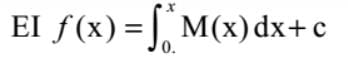Where E is the young's modulus (in Pasual, Pa), I is the second moment of area (in m4). Y is the traverse displacement of the beam x and M(x) is the bending moment at x. The SI unit of flexural rigidity is thus Pa. m4 or Nm4
∴ Dimension is ML3T–2

Practice Test: Civil Engineering (CE)- 1 - Question 16

The superelevation needed for a vehicle travelling at a speed of 60 kmph on a curve of radius 128 m on a surface with a coefficient of friction of 0.15 is:

Detailed Solution for Practice Test: Civil Engineering (CE)- 1 - Question 16

A Correct the options by inserting a zero after the decimal in each option.

e- superelevation; f- coefficient of friction = 0.15; v= speed of vehicle in m/s = 60 kmph = 60x0.278 m/s = 16.68 m/s; R = radius of curvature = 128m
ⅇ+f=v2/gR  ⅇ+0.15=〖16.68〗2/(9.81 x 128)  e= 0.0716

Practice Test: Civil Engineering (CE)- 1 - Question 17

Which of the following raingauge gives a plot of the accumulated rainfall against the elapsed time?

Detailed Solution for Practice Test: Civil Engineering (CE)- 1 - Question 17

Weighing bucket type

Practice Test: Civil Engineering (CE)- 1 - Question 18

If the time period between centroid of the rainfall diagram and peak of the hydrograph is 5 hour, using Snyder’s equation the value of the base width of unit hydrograph in hours is?

Detailed Solution for Practice Test: Civil Engineering (CE)- 1 - Question 18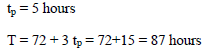Practice Test: Civil Engineering (CE)- 1 - Question 19

A river 5 m deep consists of a sand bed with saturated unit weight of 20 kN/m3 . vw = 9.81 kN/m3. The effective vertical stress at 5 m from the top of sand bed is:

Detailed Solution for Practice Test: Civil Engineering (CE)- 1 - Question 19

Effective Vertical Stress = Total Vertical Stress – Pore Water Pressure
= γ_(sat ) z - γ_(w ) z = 20x 5 – 9.81 x 5 = 50.95 kN/m2

Practice Test: Civil Engineering (CE)- 1 - Question 20

A hydraulic model of a spillway is constructed with a scale 1:16. If the prototype discharge is 2048 cumecs, then the corresponding discharge for which the model should be tested is:

Detailed Solution for Practice Test: Civil Engineering (CE)- 1 - Question 20

Q = A×V
Spillway model obeys Froude’s Law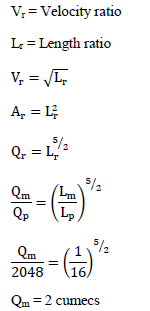Practice Test: Civil Engineering (CE)- 1 - Question 21

A droplet of water at 200C (σ = 0.0728 N/m) has internal pressure 1 kPa greater than that outside it, its diameter is nearly:

Detailed Solution for Practice Test: Civil Engineering (CE)- 1 - Question 21

For a droplet;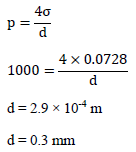Practice Test: Civil Engineering (CE)- 1 - Question 22

A reinforced concrete structure has to be constructed along a sea coast. The minimum grade of concrete to be used as per IS : 456-2000

Detailed Solution for Practice Test: Civil Engineering (CE)- 1 - Question 22

Minimum Grade of Concrete on Sea Cost:
Plain Cement Concrete – M20
Reinforced Cement Concrete – M30

Practice Test: Civil Engineering (CE)- 1 - Question 23

The order and degree of the differential equation given, is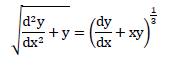Detailed Solution for Practice Test: Civil Engineering (CE)- 1 - Question 23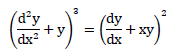Order = 2
Degree = 3

Practice Test: Civil Engineering (CE)- 1 - Question 24

The probability distribution taken to represent the completion time in PERT analysis is:

Detailed Solution for Practice Test: Civil Engineering (CE)- 1 - Question 24

The distribution curve for the time taken in to complete each activity of a project resembles a beta-distribution curve and the distribution curve for the time taken to complete entire project ( consisting of several activities) in general resembles a normal distribution curve.

Practice Test: Civil Engineering (CE)- 1 - Question 25

Consider the following statements
1) Cambium layer is between sapwood and heartwood
2) Heartwood is otherwise termed as deadwood
3) Timber used for construction is obtained from heartwood
Which of the following is/are correct statements?

Detailed Solution for Practice Test: Civil Engineering (CE)- 1 - Question 25

Cambium layer is between inner bark and sapwood. Heartwood is dead wood which does not take part in the growth of tree and is used for construction.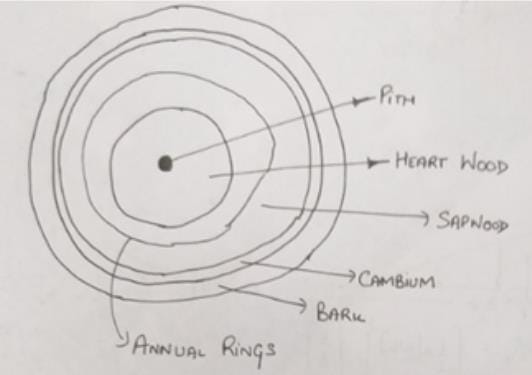Practice Test: Civil Engineering (CE)- 1 - Question 26

The true bearing of a tower T as observed from station A was 3560 and the magnetic bearing of the same was 40.The back bearing of the line AB when measured with prismatic compass was found to be 2960.Then the true fore bearing of line AB will be _____ degrees.

Detailed Solution for Practice Test: Civil Engineering (CE)- 1 - Question 26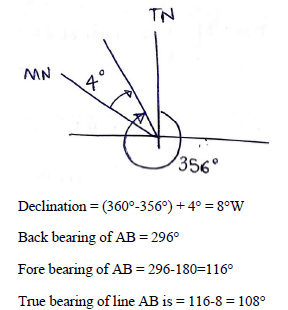Practice Test: Civil Engineering (CE)- 1 - Question 27

The degree of static indeterminacy of the rigid frame having 2 internal hinges is as shown in the figure below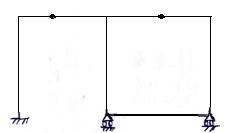Detailed Solution for Practice Test: Civil Engineering (CE)- 1 - Question 27

Number of restraints required = 6
Number of cuts required = 3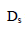= 3×3-6 = 3

Practice Test: Civil Engineering (CE)- 1 - Question 28

If the deformations of the truss-members are as shown in parentheses, the rotation of the member bd is: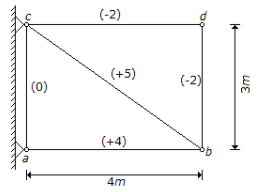Practice Test: Civil Engineering (CE)- 1 - Question 29

The problem of lateral buckling can arise only in those steel beams which have:

Detailed Solution for Practice Test: Civil Engineering (CE)- 1 - Question 29

Lateral buckling occurs when an applied load causes lateral displacement of a member which generally occurs when moment of inertia about the bending axis smaller than the other.

Practice Test: Civil Engineering (CE)- 1 - Question 30

The available moisture holding capacity of soil is 15cm per meter depth of soil. If a crop with a root zone of 0.8m and consumptive use of 6 mm/day is to be grown, the frequency of irrigation for restricting the moisture depletion to 60% of available moisture is?

Detailed Solution for Practice Test: Civil Engineering (CE)- 1 - Question 30

Available moisture holding capacity = 15×0.8 = 12cm
Readily available moisture content = 0.4×12 = 4.8cm
Frequency of irrigation = 48/6 = 8 days

Practice Test: Civil Engineering (CE)- 1 - Question 31

6 boys and 6 girls sit in a row at random. Find the probability that-
1) The six girls sit together
2) The boys and girls sit alternately.

Detailed Solution for Practice Test: Civil Engineering (CE)- 1 - Question 31

\Total ways of making sit 12 persons  12!
Considering set of 6 girls as a single group. Within the group girls can be arranged in 6! Ways. Now only among 7 persons—> 6 boys + 1 girl group arrangement needs to be done. Thus, number of ways for the same = 7!.
Therefore, probability = Favourable outcomes/ total number of outcomes = (7!) (6!) / (12!) = 1/1322
We can only make sit boys and girls in alternate manner in two possible ways, boys at even and girls at even. But, among boys group and girls group itself, rearrangement can be done in 6! Ways each.
Therefore, probability =(2)(6!)(6!)/ 12! = 1/462

Practice Test: Civil Engineering (CE)- 1 - Question 32

Match List-I (contract types) with List-II (Characteristics):
List-I
a. Labour contract
b.Item rate contract
c. Piecework contract
d.Lump sum contract
List-II
1.Petty works and regular maintenance work
3.Payment made by detailed measurement of different time
4.Not practiced in government

Detailed Solution for Practice Test: Civil Engineering (CE)- 1 - Question 32

Labour Contract- Workers who are hired for a specific task and a finite period.
Item Rate Contract- Contractor gets paid on ‘per item basis’-Payment by detailed measurement of different time.
Piecework Contract- Paying contractor pay rate for each unit/ work they produce/accomplish-Petty works and regular maintenance work
Lump sum Contract-A single lump sum price for all works is agreed before works begin. Adopted for buildings, roads, bridges and electrical works.

Practice Test: Civil Engineering (CE)- 1 - Question 33

Plate bearing test with 20 cm diameter plate on soil subgrade yielded a pressure of 1.25 x 105 N/m2 at 0.5 cm deflection. What is the elastic modulus of subgrade?

Detailed Solution for Practice Test: Civil Engineering (CE)- 1 - Question 33

For Rigid Plate
Δ=(1⋅18pa)/Es  0.005 =(1⋅18 x 1.25 x 105 x 0.1 )/Es   Es = 29.5 x 105 N/m2
Δ – Settlement = 0.5 cm = 0.005 m
P – pressure = 1.25 x 105 N/m2
radius of plate = (20/2) cm =10 cm = 0.1m

Practice Test: Civil Engineering (CE)- 1 - Question 34

A ductile metal bar is subjected to pure shear test and its yield strength was found to be 230 N/mm2 the maximum permissible shear stress on that metal will be:

Detailed Solution for Practice Test: Civil Engineering (CE)- 1 - Question 34

fy = 230 kN/m2
Shear strength = 230/2 = 115 N/mm2
When ductile metal bar is subjected to pure shear test, maximum permissible shear stress is 115N/mm2

Practice Test: Civil Engineering (CE)- 1 - Question 35

Which of the following is characteristic of colliform organism?
1.Spore formation
2.Gram negative
3.Gram positive
4.Lactose fermenter
5.Bacillus
Select the correct answer using the codes given below:

Detailed Solution for Practice Test: Civil Engineering (CE)- 1 - Question 35

Colliform organism is bacillus and gives gram negative test. It is lactose fermenter.

Practice Test: Civil Engineering (CE)- 1 - Question 36

A light rope fixed at one end of a wooden clamp on the ground passes over a tree branch and hangs on the other side. It makes an angle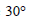with the ground. A man weighing (60 kg) wants to climb up the rope. The wooden clamp can come out of the ground if an upward force greater than 360N is applied to it. Find the maximum acceleration in the upward direction with which the man can climb safely. Neglect friction at the tree branch. Take g = 10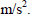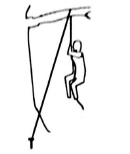Detailed Solution for Practice Test: Civil Engineering (CE)- 1 - Question 36

Let T be the tension in the rope.
The upward force on the clamp is, T sin= T/2
The maximum tension that will not detach the clamp from the ground is:
T/2 =360N
T = 720 N
T – 600 = 60a
where a is the acceleration of man in upward direction.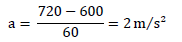Practice Test: Civil Engineering (CE)- 1 - Question 37

A pavement designer has arrived at design traffic of 100 million standard axles for a newly developing national highway as per IRC : 37 guidelines using the following data: design life = 15 years, commercial vehicle count before pavement construction = 4500 vehicles/day, annual traffic growth rate = 8%. The vehicle damage factor used in the calculation was

Detailed Solution for Practice Test: Civil Engineering (CE)- 1 - Question 37

The cumulative standard axles may be calculated as

NS=365×A×[(1+r)n−1)]×F×Dr

Ns – Cumulative standard axles in standard axles

A – Number of the commercial vehicle per day when pavement construction is completed

r – Rate of growth of traffic

n – Design life of the pavement

F – Vehicle damage factor

D – Lane distribution factor

Calculation:

Given

A = 4500 vehicles/ day

r = 8%

Ns = 100 × 106

n = 15 years

100×106=365×4500×[(1+0.08)15−1)]×F0.08

Solving we get F = 2.24

Practice Test: Civil Engineering (CE)- 1 - Question 38

The present population of a community is 28000 with an average water demand of 150 Lpcd. The existing water
treatment plant has a design capacity of 6000 m3/d. It is expected that population will increase to 48,000 in the next 20 years. What is the number of years from now when the plant will reach its design capacity assuming an arithmetic rate of population growth?

Detailed Solution for Practice Test: Civil Engineering (CE)- 1 - Question 38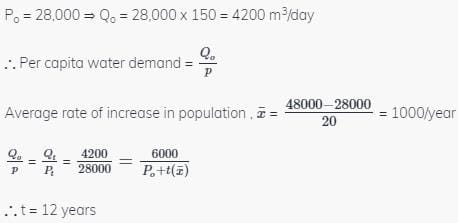Practice Test: Civil Engineering (CE)- 1 - Question 39

A square plate 5m × 5m hangs in water from one of its corners and its centroid lies at 8m from the free water surface. Find the total pressure force on the top half of the plate. (γw = 10 kN/m3

Detailed Solution for Practice Test: Civil Engineering (CE)- 1 - Question 39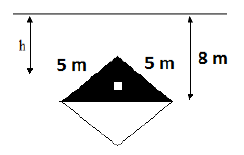Pressure force = Pressure at CG × Area of plate
h is the height of CG from top of water surface.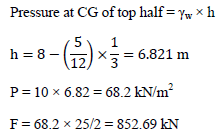Practice Test: Civil Engineering (CE)- 1 - Question 40

A sample of clay was coated with paraffin wax and its mass, including the mass of wax was found to be 650 gm, the sample was immersed in water and the volume of the water displaced was found to be 325 Ml. The mass of the sample without wax was 640 gm and water content of the specimen was 20%. Specific gravity of wax is 0.9 and specific gravity of soil solids is 2.7. The void ratio and density of the sample is:

Detailed Solution for Practice Test: Civil Engineering (CE)- 1 - Question 40

Mass of wax is 650-640 = 10 gm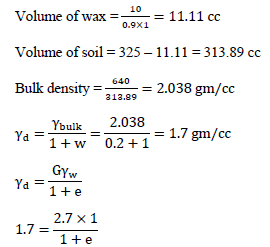e = 0.588

Practice Test: Civil Engineering (CE)- 1 - Question 41

For a highway with design speed of 100 kmph, the safe overtaking sight distance is (assume accelerations as 0.53 m/sec2)

Detailed Solution for Practice Test: Civil Engineering (CE)- 1 - Question 41

V = 100kmph, Vb = V - 16 = 100 - 16 = 84kmph

Take, t = reaction time = 2secs

A = 0.53 m/s2 = 0.53 * 36 = 1.92 kN/s2

Now d1 = 0.28 * Vb * t = 0.28 * 84 * 2 = 47.04m

d2 = 0.28Vb * T; T = ; s = 0.2Vb + 6 = 0.28 * 84 + 6 = 22.8m

then T = 13.11secs

d2 = 0.28 * Vb * T = 0.28*84*13.11 = 354.13m

d3 = 0.28V * T = 0.28 * 100 * 13.11 = 367.08m

d1 +d2 + d3 = 768.25m = 750m

Practice Test: Civil Engineering (CE)- 1 - Question 42

The insitu moisture content of a soil is 18% and its bulk unit weight is 19.65 kN/m3 .The specific gravity of the soil solids is 2.7. The soil is to be excavated and transported to a construction site and then compacted to a minimum dry unit weight of 16.65  kN/m3 at a moisture content of 20%. How many truck loads are needed to transport the excavated soil if each truck can carry 25 tons? Total volume of the compacted fill is 10,000 m3 (γw = 10 kN/m3)

Detailed Solution for Practice Test: Civil Engineering (CE)- 1 - Question 42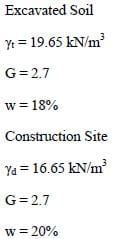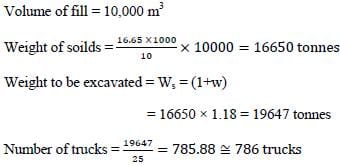Practice Test: Civil Engineering (CE)- 1 - Question 43

Horizontal stiffness coefficient, K11 of bar ab is given by: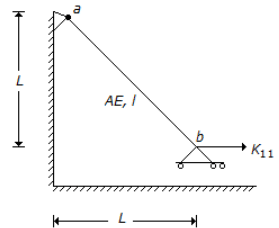Detailed Solution for Practice Test: Civil Engineering (CE)- 1 - Question 43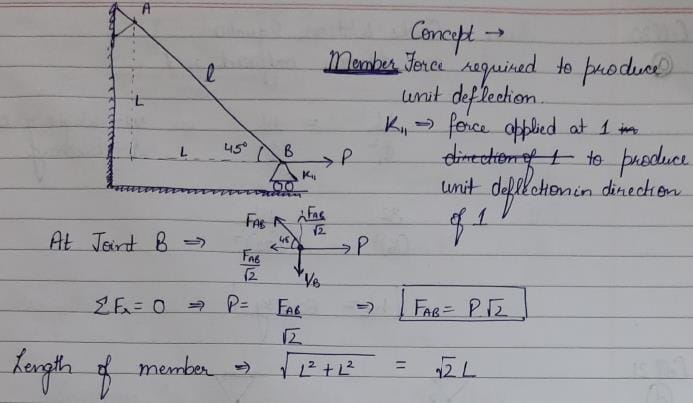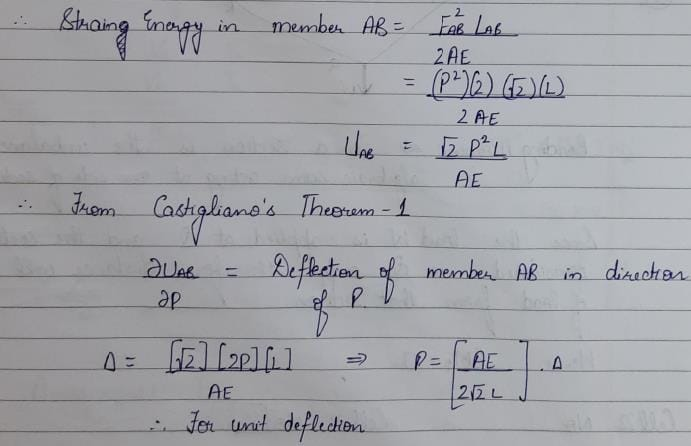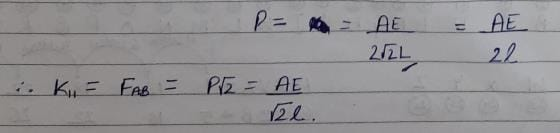Practice Test: Civil Engineering (CE)- 1 - Question 44

In the given figure below, the slope at point P will be: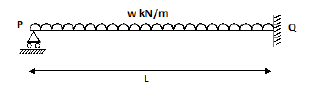Detailed Solution for Practice Test: Civil Engineering (CE)- 1 - Question 44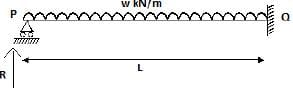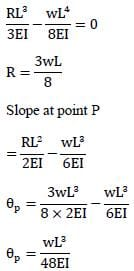Practice Test: Civil Engineering (CE)- 1 - Question 45

A father notes that when his teenage daughter uses the phone she takes no less than 6 minutes for a call but sometimes as much as an hour. 20 minutes calls are more frequent than calls of any other duration.If the daughter’s calls were to be represented as an activity in PERT project, the expected duration of each phone call is:

Detailed Solution for Practice Test: Civil Engineering (CE)- 1 - Question 45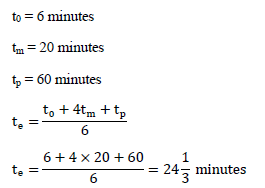Practice Test: Civil Engineering (CE)- 1 - Question 46

Calculate the quantity of cement (in kg) required to produce 1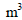of concrete for mix proportion of 1:2:4 (by volume). Water cement ratio = 0.48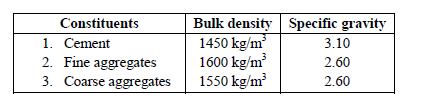Percentage of entrapped air is 2%

Detailed Solution for Practice Test: Civil Engineering (CE)- 1 - Question 46

Let the volume of cement, fine aggregates and coarse aggregates be x, 2x and 4x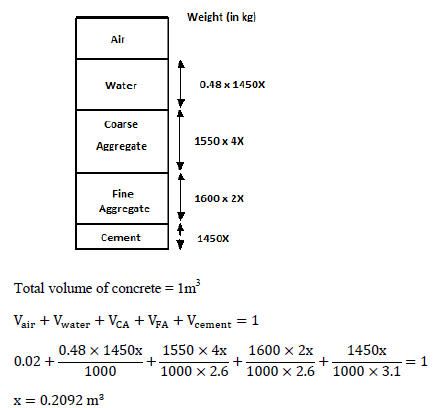Weight of cement added = 1450 × 0.2092 = 303.4 kg

Practice Test: Civil Engineering (CE)- 1 - Question 47

The beams in the two storey frame shown in the figure below have a cross – section such that the flexural rigidity may be considered infinite. Which among the following is the stiffness matrix for the structure in respect of the global coordinates 1 and 2?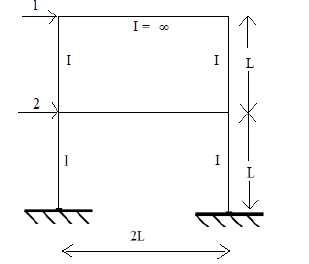Detailed Solution for Practice Test: Civil Engineering (CE)- 1 - Question 47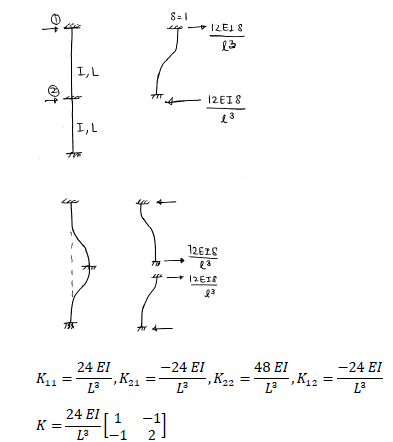Practice Test: Civil Engineering (CE)- 1 - Question 48

A uniformly distributed load of 6 kN/m, 6m long crosses a girder 24m long. What is the maximum bending moment at a section 8m from the right end in a simply supported girders?

Detailed Solution for Practice Test: Civil Engineering (CE)- 1 - Question 48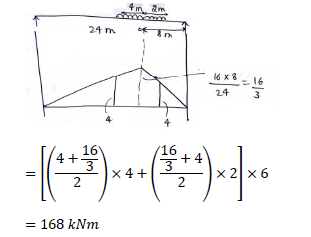Practice Test: Civil Engineering (CE)- 1 - Question 49

The staff reading taken on a staff held at 200m from the instrument. The division of bubble tube is 2.5mm. Following observations were made.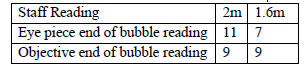The radius of curvature of bubble will be ________m

Detailed Solution for Practice Test: Civil Engineering (CE)- 1 - Question 49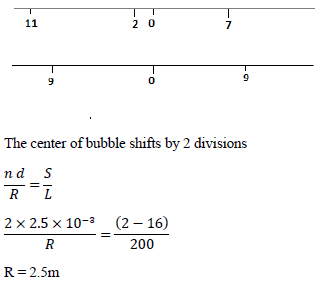Practice Test: Civil Engineering (CE)- 1 - Question 50

Vertical reaction developed at B in frame below due to applied load of 100 kN (with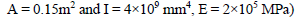is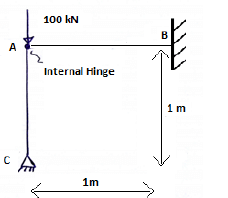Detailed Solution for Practice Test: Civil Engineering (CE)- 1 - Question 50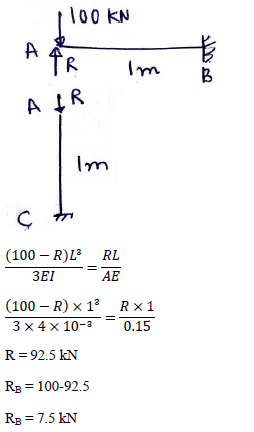Practice Test: Civil Engineering (CE)- 1 - Question 51

The maximum permissible deflection for a gantry girder, spanning over 6 m, on which an EOT (electric overhead travelling) crane of capacity 200 kN is operating, is

Detailed Solution for Practice Test: Civil Engineering (CE)- 1 - Question 51

Maximum vertical deflection of Gantry girder for EOT of capacity less than 500KN is ( L/750), where L in mm
For EOT having capacity more then 500KN deflection is (L/1000) and for manually operated crane Deflection is (L/500)

Practice Test: Civil Engineering (CE)- 1 - Question 52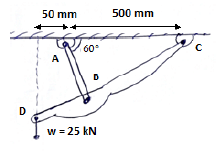A rectangular link AB made of steel is used to support a load W through a road CD as shown in the figure. If the link AB is 30mm wide, determine its thickness for a factor of safety 2.5. The ultimate strength of steel may be assumed to be 450 MPa.

Detailed Solution for Practice Test: Civil Engineering (CE)- 1 - Question 52

Drawing Free body diagram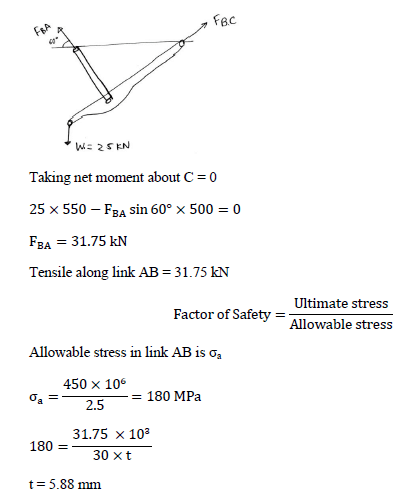Practice Test: Civil Engineering (CE)- 1 - Question 53

An RCC beam of size 350mm (width) and 600mm (overall depth) is subjected to shear force of 100 kN, bending moment of 200 kNm and twisting moment of 50 kNm. Effective cover to reinforcement is 25 mm. Find the equivalent bending moment which is contributed by torsional moment?

Detailed Solution for Practice Test: Civil Engineering (CE)- 1 - Question 53

Here we have to calculate the equivalent bending moment contributed by torsional moment.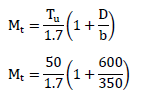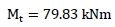Practice Test: Civil Engineering (CE)- 1 - Question 54

If the statically indeterminate beam as shown in the figure below, carries a load P at Q and a load nP at S. What is the value of n to make the structure collapse with at least 3 hinges?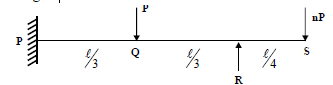Detailed Solution for Practice Test: Civil Engineering (CE)- 1 - Question 54

For atleast 3 hinges to form PR and RS span should collapse simultaneously.For PR span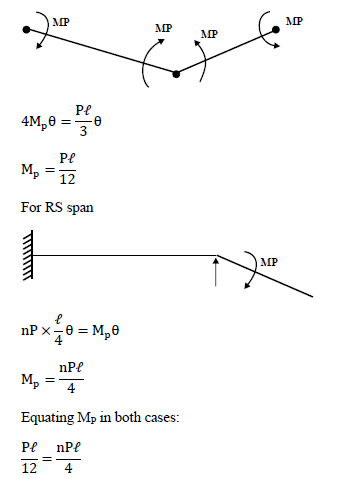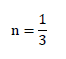Practice Test: Civil Engineering (CE)- 1 - Question 55

The length of runway at sea level, standard atmosphere condition and zero gradient is 1500m. The airport site has an elevation of 1200m and the reference temperature as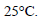It is proposed to construct a runway at an effective gradient of 2%. Find the actual runway length at the site:

Detailed Solution for Practice Test: Civil Engineering (CE)- 1 - Question 55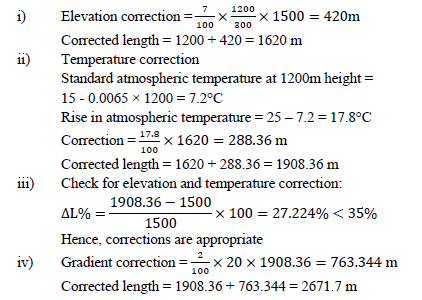Practice Test: Civil Engineering (CE)- 1 - Question 56

On sag (or valley) curves the available sight distance is determined based on:

Detailed Solution for Practice Test: Civil Engineering (CE)- 1 - Question 56

Driver can see the obstacles or vehicle which moves at either side of curvature & reduce the accident.

Practice Test: Civil Engineering (CE)- 1 - Question 57

A rectangular concrete beam of width 150mm and depth 200m is prestressed by pretensioning to a force of 200 kN at eccentricity of 30mm. The cross sectional area of prestressing steel is 200mm2 . Given Ec = 3 x 10  MPa and Est = 2 x 105 MPa. The percentage loss of stress in the prestressing steel due to elastic deformation of concrete is:

Detailed Solution for Practice Test: Civil Engineering (CE)- 1 - Question 57

P = 200 kN
E = 30mm
A = 150mm × 200mm
Stress in concrete at the level of steel bar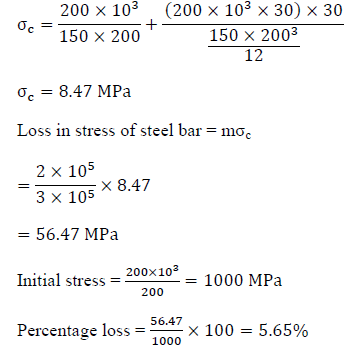Practice Test: Civil Engineering (CE)- 1 - Question 58

A portion of an embankment having a uniform up-gradient 1 in 500 is circular with radius 1000 m of the centre line. It subtends 180° at the centre. If the height of the bank is 1 m at the lower end, and side slopes 2:1, the earth work involved.

Detailed Solution for Practice Test: Civil Engineering (CE)- 1 - Question 58

For 2:1 slope top radius is 500m, and we have bottom radius is 1000m.

Volume of truncated cone is 3.14*h*((R*R) + (r*r) + (R*r))*(1/3).

= 3.14*1**((1000*1000) + (500*500) + (1000*500))*(1/3).

= 27500.

Practice Test: Civil Engineering (CE)- 1 - Question 59

The value of bearing capacity factor for cohesion, Nc, for piles as per Meyerhof, is taken as:

Detailed Solution for Practice Test: Civil Engineering (CE)- 1 - Question 59

Meyehoff bearing capacity factors for cohesive clays (phi=0) are
Nc = 5.14; Nq = 1; Ny = 0

Practice Test: Civil Engineering (CE)- 1 - Question 60

If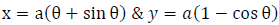,Find the value of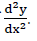Detailed Solution for Practice Test: Civil Engineering (CE)- 1 - Question 60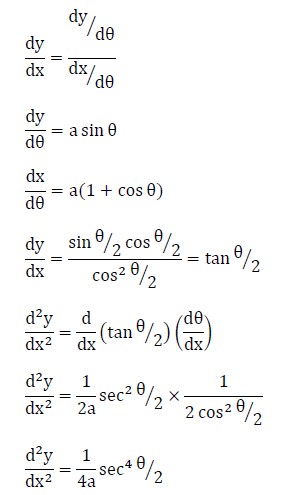Practice Test: Civil Engineering (CE)- 1 - Question 61

A trapezoidal channel with side slope of 450 is to be designed to convey a discharge of 9 m3/sec at a velocity of 1.5 m/s such that the amount of concrete lining for the bed and side is minimum. The area of lining required per meter length of channel will be?

Detailed Solution for Practice Test: Civil Engineering (CE)- 1 - Question 61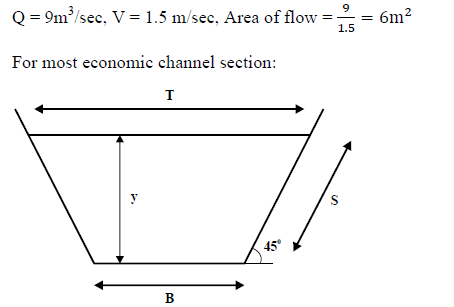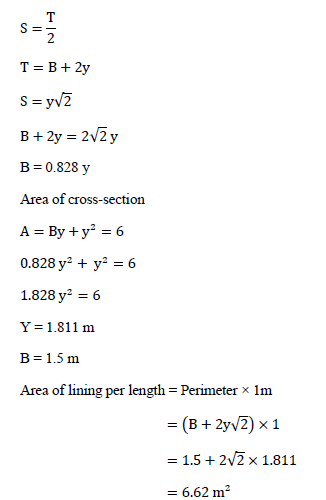Practice Test: Civil Engineering (CE)- 1 - Question 62

A man of mass m is standing on a platform of mass M kept on a smooth ice. If the man starts moving on the platform with a speed v relative to the platform, with what velocity relative to the ice does the platform recoil?

Detailed Solution for Practice Test: Civil Engineering (CE)- 1 - Question 62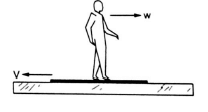v = w + V
Taking the platform and man to be a system, there is no external horizontal force on the system. The linear momentum of the system is constant.
0 = MV – mw
MV = m (v-V)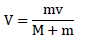Practice Test: Civil Engineering (CE)- 1 - Question 63

A 40 year flood data at a point on a river yielded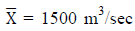and a standard deviation of 750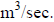For what discharge would the structure be designed to provide 90% assurance that the structure would not fail in next 60 years.

Detailed Solution for Practice Test: Civil Engineering (CE)- 1 - Question 63

Assurance = 90%
Risk = 10%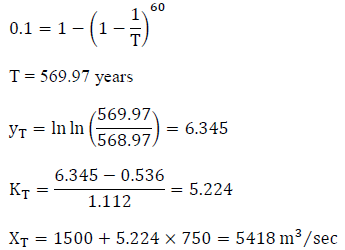Practice Test: Civil Engineering (CE)- 1 - Question 64

The left canal of tank irrigation carries a discharge of 10 cumecs and a culturable commanded area of 9000 Ha. The intensity of irrigation of Rabi crop is 70 percent and base period is 120 days. The right canal scheme carries a discharge of 24 cumecs and has a culturable commanded area of 1500 Ha. The intensity of Rabi crop is 80 percent and base period is 120 days.

Detailed Solution for Practice Test: Civil Engineering (CE)- 1 - Question 64

Left Canal: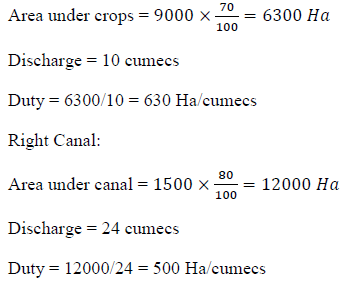Since left canal has more duty, hence left canal is more efficient than right canal

Practice Test: Civil Engineering (CE)- 1 - Question 65

A straight line AC of length 2000m is required to be set out at right angles to a given line AB. This is done by travelling from A towards C. The observations are as follows: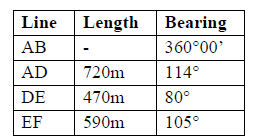Compute the necessary length of FC.

Detailed Solution for Practice Test: Civil Engineering (CE)- 1 - Question 65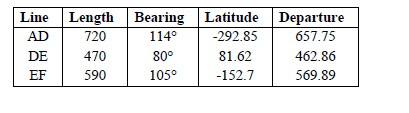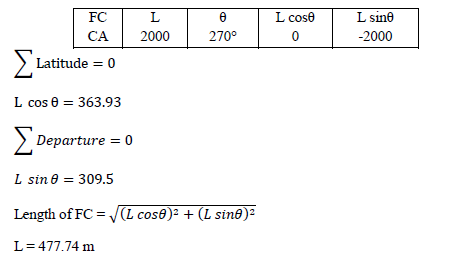## GATE Civil Engineering (CE) 2023 Mock Test Series

27 docs|296 tests
 Use Code STAYHOME200 and get INR 200 additional OFF Use Coupon Code
Information about Practice Test: Civil Engineering (CE)- 1 Page
In this test you can find the Exam questions for Practice Test: Civil Engineering (CE)- 1 solved & explained in the simplest way possible. Besides giving Questions and answers for Practice Test: Civil Engineering (CE)- 1, EduRev gives you an ample number of Online tests for practice

## GATE Civil Engineering (CE) 2023 Mock Test Series

27 docs|296 tests